Operator IntParamTranslator

Operator IntParamTranslator allows translated access (read and write) to parameters of one or several other operators. For calculation, you define formulas. The syntax for these formulas complies to GenICam API version 2.0. A detailed syntax description you find below.

Operator IntParamTranslator generates a map parameter of type VA_SINT. The name of the map parameter is Value.

Although the operator defines the type of its parameter Value to be of type VA_SINT, it does not restrict the data types of the parameters which are accessed during the write or read operations. For example, the formulas defined in the operator can contain access to parameters of type float.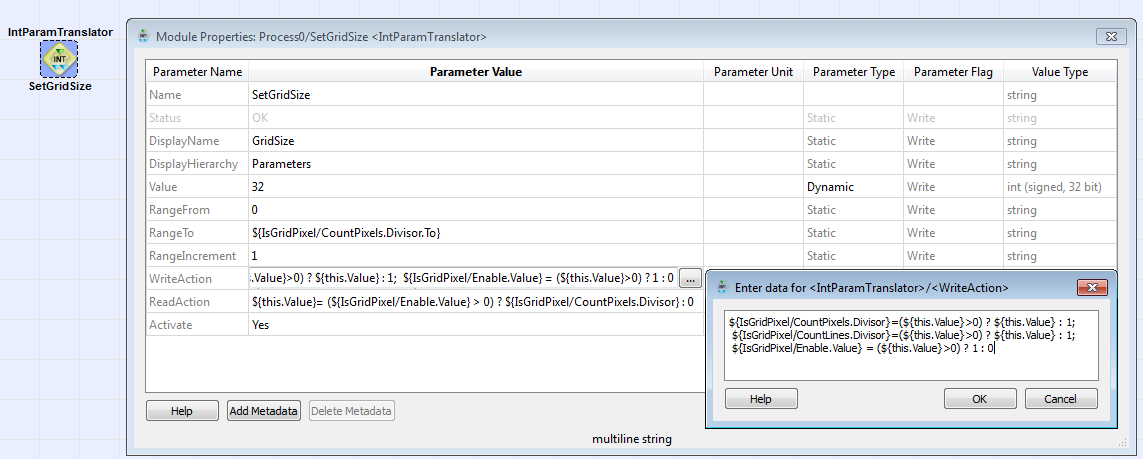The formula can contain values of operator parameters anywhere in the design. As soon as one of these values changes, the calculation of translation operator's parameter Value will end up with a new result (different to the value parameter Value held before). The formula can not only incorporate the values of parameters, but also specific properties of parameters, such as minimal value, maximal value, step size, or the numerical value of enumeration items.

Write Action

In parameter WriteAction, you define what happens during a write access to parameter Value of the translation operator. In contrast to using the reference operators, you do not simply forward the value of parameter Value to another parameter in the design. Instead, the value of parameter Value is used for a set of write actions you define in parameter WriteAction of the translation operator. Write actions are composed of one or several equations. On the left-hand side of these equations you define which parameter of which operator receives the result of the calculation. If you define more than one equation, separate the equations via semicolon. As soon as the there happens a write access to parameter Value, the formula(s) on the right-hand side of the equations you defined in parameter WriteAction is/are carried out and the result(s) is/are forwarded to the parameters specified on the left-hand side of the equations.

The formula can refer to values of operator parameters anywhere in the design. As soon as one of these values changes, the calculation results will differ when you re-write the same value as before to the Value parameter. The formula can not only incorporate the values of parameters, but also specific properties of parameters, such as minimal value, maximal value, step size, or the numerical value of enumeration items.

Formulas for Calculation of Value Range

You can also add formulas to calculate the current value range of parameter Value. You define the according formulas in the parameters RangeFrom, RangeTo, and RangeIncrement (step size). The formulas can contain values of module parameters anywhere in the design, as well as specific properties of these parameters, such as minimal value, maximal value, step size, or the numerical value of enumeration items.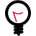Formula Syntax: Mathematical Operations

The syntax complies to the GenICam API standard in version 2.0. The allowed formula elements are identical to the formula elements defined in the GenICam standard:

 Basic operations: () brackets + - * / addition, subtraction, multiplication, division % remainder ** power & | ^ ~ bitwise: and / or / xor / not <> = > < <= >= logical relations: not equal / equal / greater / less / less or equal / greater or equal && || logical and / logical or << >> shift left / shift right

Table 39. Basic operations

Conditional operator

<condition> ? <true_expr> : <false_expr>

Example:

\${target.Value} = (\${this.Value} > 0) ? 1 : 0;

 Functions: SGN(x) return sign of x. Returns +1 for positive argument and -1 for negative argument NEG(x) swap sign of x ABS(x) return absolute value of x SQRT(x) return square root of x TRUNC(x) truncate x, which means returning the nearest integral value that is not larger in magnitude than x FLOOR(x) Round downward, returning the largest integral value that is not greater than x CEIL(x) Round upward, returning the smallest integral value that is not less than x ROUND(x,precision) round x to the number of decimal fractional digits given by precision, with halfway cases rounded away from zero SIN(x) return sine of an angle of x radians COS(x) return cosine of an angle of x radians TAN(x) return the tangent of an angle of x radians ASIN(x) return the principal value of the arc sine of x, expressed in radians ACOS(x) return the principal value of the arc cosine of x, expressed in radians ATAN(x) return the principal value of the arc tangent of x, expressed in radians EXP(x) return the base-e exponential function of x, which is e raised to the power x: ex LN(x) return the natural logarithm of x The natural logarithm is the base-e logarithm: the inverse of the natural exponential function (exp). LG(x) return the common (base-10) logarithm of x E() return Euler's number, 2.7182818284590451 PI() return circle constant, 3.1415926535897931

Table 40. Functions

Example:

\${target.Value} = NEG(\${this.Value})

GenICam Support for Individual Functions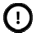With access via GenICam, support for funtions SGN(x) and NEG(x) is guaranteed. The other functions mentioned above may or may not be supported by a specific GenICam implementation. This is of special interest to eVA platforms using the GenICam interface.Paths to Parameters

To access an operator parameter any place within your design, you need to provide the path to this parameter in your formula.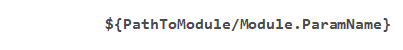"PathToModule": Here, you define the relative path to the operator who's parameter you want to access. The path is relative to the hierarchical level the translation operator itself is located. You also define the name of the accessed operator. Use a slash as hierarchy separator.

"Module": Name of module.

As name for the translation operator instance itself you use the name "this".

Keep your Modules IndependentIt is not allowed to define a path towards a hierarchical level higher than the hierarchical level the translation operator is located. This rule follows the logic that a hierarchical module is not allowed to know anything about the environment it is instantiated in, because only in this case it can be used as a freely relocatable and replicable module.
 Access to Parameter PropertiesThe formulas can not only incorporate the values of parameters, but also specific properties of parameters, such as minimal value, maximal value, or step size: \${PathToModule/Module.ParamName.From} or \${PathToModule/Module.ParamName.Min}: minimal valid value of parameter PathToModule/Module.ParamName. \${PathToModule/Module.ParamName.To} or \${ PathToModule/Module.ParamName.Max}: maximum valid value of parameter PathToModule/Module.ParamName. \${PathToModule/Module.ParamName.Inc}: Increment (step size) between two valid values of parameter PathToModule/Module.ParamName. \${PathToModule/Module.ParamName.Enum("EnumName")}: Integer value of enumeration name EnumName.
 Syntax for Write Access EquationsEquations for write actions you define in parameter WriteAction. They have the following syntax: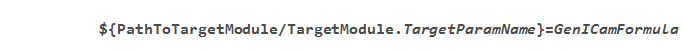Here, you can define multiple equations for multiple target parameters. Use a semicolon as separator between the individual equations. "this" refers to the translation operator instance itself. Example for a WriteAction Equation: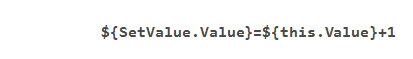Example: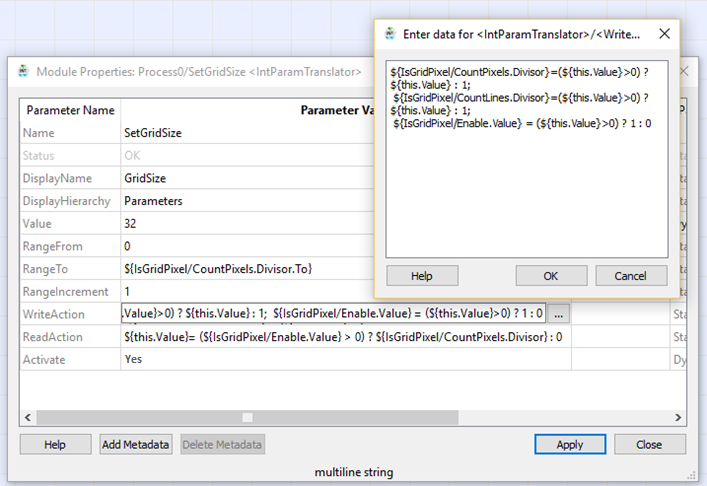Syntax for Read Access EquationThe equation for the read action you define in parameter ReadAction. The equation always starts with "\${this.Value}=" . It has the following syntax: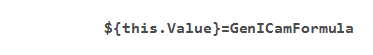Example for a ReadAction equation: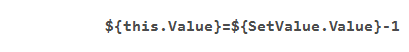Referencing Map Parameters

You can define a target within the design hierarchy where map parameter Value will be visible and accessible. The target is defined by two parameters: DisplayHierarchy and DisplayName. In DisplayHierarchy you address a hierarchical box within the same process the translation operator itself is located in. In parameter DisplayName you set up the name for the target parameter. If DisplayHierarchy and DisplayName are both set, the parameter defined by DisplayName will be available in the hierarchical box addressed by DisplayHierarchy. If DisplayName is empty, no parameter is created. If DisplayName is set, but DisplayHierarchy is empty, the parameter defined by DisplayName is added to the parent hierarchy (i.e., the hierarchical box the translation operator itself is located in).

This allows you to make map parameter Value accessible on any hierarchical level (of the design) you want. This is especially helpful when workin with protected hierarchical boxes. You can make the parameter available directly under the properties of the protected hierarchical box itself.

Mapped parameters can be referenced themselves. Thus, you can reference either the content of parameter Value in a translation operator, or reference the parameter you have defined in the parameters DisplayName and DisplayHierarchy. This is especially important when protected hierarchical boxes are built up out of other protected hierarchical boxes.

The operator has no inputs and outputs, as it doesn't interfere with the data flow structure of the design.

A general introduction into library Parameters you find in Library Parameters.

I/O Properties

Property Value
Operator Type None (since there are no inputs or outputs)

None

Parameters

Status
Type static read (although the GUI displays write) parameter
Default none
Range OK or error message

Displays the error status. If parameter Activate is set to Yes, the other operator settings are checked. This parameter displays the result of this check, i.e., either OK or an error message.

This parameter is not part of the final applet.

DisplayName
Type static write parameter
Default none
Range any string

If you want to grant access from and to the parameter Value at another point in the design, you need to define a new parameter. Specify here the name of this new parameter.

If this field is empty, no new parameter is created.

DisplayHierarchy
Type static write parameter
Default none
Range any string

Specify here the hierarchical box to which you want to attach the new map parameter you defined in parameter DisplayName.

If you defined a new parameter DisplayName, but leave parameter DisplayHierarchy empty: The new parameter is added to the hierarchical box that contains the reference operator instance. (Keep in mind this is not possible if the reference operator instance is located on the highest (process) level.)

Syntax for Setting up Path to Display Target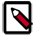If you want the new parameter to show up in another place of the design, you need to specify the path to the hierarchical box that is to contain the new parameter in parameter DisplayHierarchy. The syntax is as follows: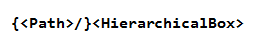is the relative path from process level to the hierarchical level of the design where the is located that is to receive the new parameter. Thus, parameter DisplayHierarchy can only address hierarchical boxes within the same process. Use a slash as separator between different hierarchical levels. is the name of the hierarchical box that is to receive the new map parameter. Example: Level0/Level1
Value
Type static, dynamic, write, read parameter
Default 0
Range Signed integer (64 bit)

Unit
Type static write parameter
Default -
Range Latin-1

Enter here the unit for the parameter Value.

Description
Type static write parameter
Default -
Range any text

Enter here the description for the parameter Value.

RangeFrom
Type static write parameter
Default 0
Range

Definition of smallest valid value. You can also enter a formula here.

RangeTo
Type static write parameter
Default 255
Range

Definition of biggest valid value. You can also enter a formula here.

RangeIncrement
Type static write parameter
Default 1
Range

Definition of intervall between valid values (definition of stepsize). You can also enter a formula here.

WriteAction
Type static write parameter
Default
Range

Type static write parameter
Default
Range

The equation always starts with "\${this.Value}=" .

Activate
Type dynamic write parameter
Default No
Range {No,Yes}

No = Access to and from referenced parameters (via read and write equations) is de-activated and parameter Status disabled. Parameters DisplayName and DisplayHierarchy have no effect.

Examples of Use

The use of operator IntParamTranslator is shown in the following examples:

• 'Hardware Test'

An example for hardware self test of DMA, RAM, GPIOs, Trigger and LEDs.

• 'Parameter Translation'

Examples - Demonstration how to use the parameter translation operators for manipulation of parameters.